# Excel Training: Formulas

## Calculation formulas

To write a formula, start with a `=`, then add a number, then an operator (for example `+`), then another number, and so on, adding `( )` when necessary.

For example:

`=50+15` (the displayed result will be: 65)

`=(50+15)^2/5` (the displayed result will be: 845)

Here is a list of operators useful for your calculations:

OperatorOperationExample of formulaResult
-Subtraction=10-55
*Multiplication=10*550
/Division=10/52
^Exponent=10^5100000

You now know how to write a formula by directly entering the numbers into the formula. But in most cases, you will need to enter references to cells containing these numbers instead of directly entering the numbers.

The following example will help you understand the usefulness of entering references to cells instead of numbers.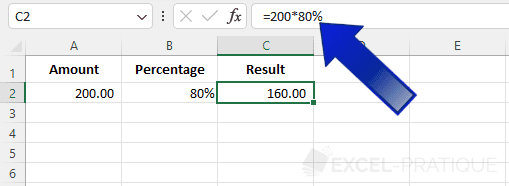As you can see in the formula displayed just above, the numbers were entered directly into the formula `=200*80%`.

The problem with this method is that if you now change the values in cells A2 or B2, you will have to remember to modify the formula with each change, which is really not practical: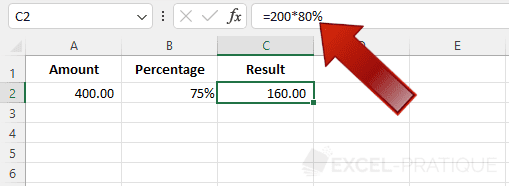If, on the other hand, you enter references to cells instead of entering the numbers in the formula, the problem no longer arises. The formula then becomes `=A2*B2`: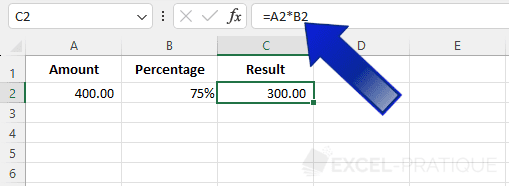By doing this, when you modify cells A2 or B2, the result of the formula will automatically be recalculated: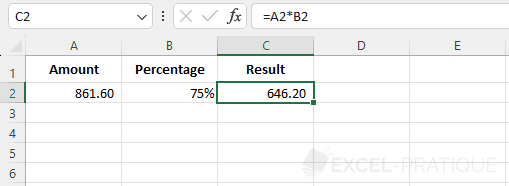To add a reference to a cell, click on the relevant cell (after entering `=`) or directly type its reference into the formula.

## Copying formulas

As we saw in the previous lesson, copying also works very well with formulas: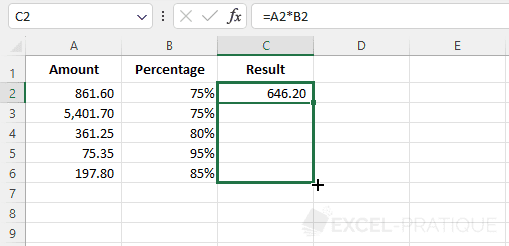The starting formula (on line 2) is `=A2*B2` and after copying, the formula on line 6 is `=A6*B6`: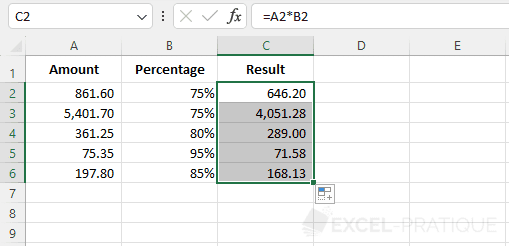The cell references have been shifted according to the direction of the copy, but in some cases, a fixed cell reference will be needed...

To illustrate this, the amount in cell B9 now needs to be divided into 3 parts. The formula becomes `=A2*B2/B9` and this is then copied: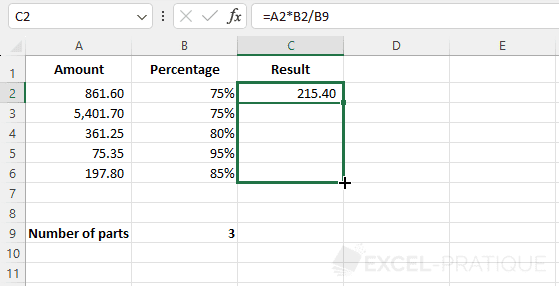Contrary to appearances, the copy worked quite normally: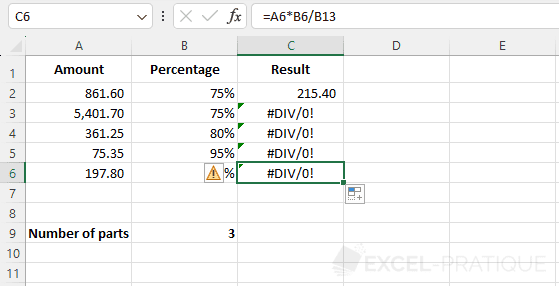The problem that arises here is that (you can see it by double-clicking on cell C6) cell B9 has been shifted during the copy: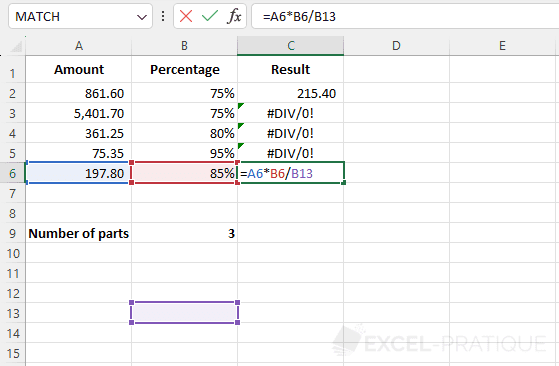To avoid this, the reference must be fixed by adding \$ to the reference.

The formula `=A2*B2/B9` then becomes `=A2*B2/\$B\$9`: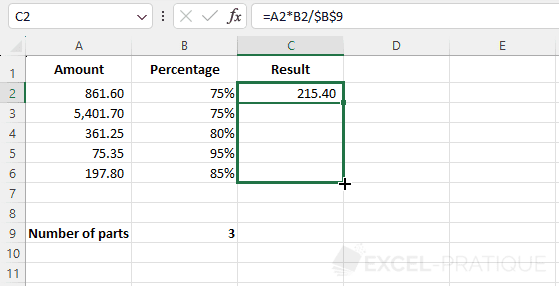All formulas now refer to cell B9: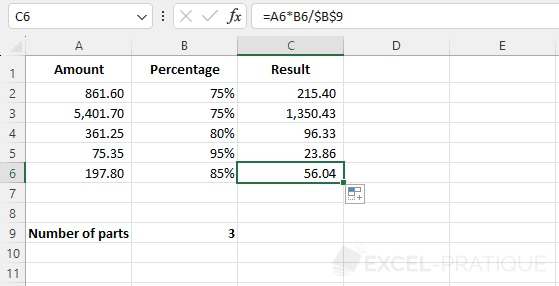The first \$ of \$B\$9 fixes the column when copying and the second \$ the row. In this example, it is therefore possible to fix only the row with B\$9 since there is no column shift when copying.